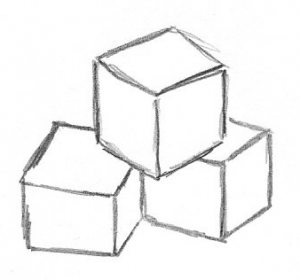# 3After posting about Booker and Sutherland’s cool expression of 42 as a sum of three cubes, Drew Sutherland wrote to say that they found a new way to write 3 as a sum of three cubes:

$569936821221962380720^3 + (-569936821113563493509)^3 + (-472715493453327032)^3 = 3$.

As explained below, this is both amazing and predicted.  Some earlier computer attempts turned up no new solutions from what Mordell had already found.  Nevertheless, Heath-Brown expected an infinite number of solutions, and even estimated their density.  However, it wasn’t until Booker and Sutherland had cracked the much harder nuts 33 and 42 that this new solution for 3 was found.  It sure helps to have access to half a million cores!

Sutherland gave me permission to quote his message, which tells the latest story well.

[Note: I made minor edits, including some formatting to work in WordPress.]

---- Begin included message ----

While this might not be as exciting to Douglas Adams fans, it arguably has more mathematical significance, given the history of the problem. As Mordell wrote in 1953 [1,p.505],

“I do not know anything about the integer solutions of beyond the existence of the four sets (1, 1, 1), (4, 4, —5) etc.; and it must be very difficult indeed to find out anything about any other solutions*

This spurred several unsuccessful searches for other solutions to $x^3 +y^3 + z^3 = 3$, including [2,3], which led some to speculate that there were no further solutions.  But in 1992 Heath-Brown conjectured that there should be infinitely many, and estimated that the density of such solutions was such that the next expected solution was simply beyond the range that had been searched to that point, writing [4,p.623]:

“A search for solutions to these equations (Gardiner, Lazarus, and Stein ) found only (1, 1, 1) and (4,4,-5), corresponding to $k = 3…$ Indeed, it was suggested that these equations have no more solutions. Inasmuch as the search range was only to $2^{16} \sim 6.5 \times 10^4$, the figures above indicate that any such conjecture is premature.”

Heath-Brown’s calculations suggested that one might expect the next solution to satisfy max(|x|,|y|,|z|) < 10^8, but later searches [5,6,7] yielded no new solutions even with $\max(|x|,|y|,|z|) < 10^{15}$.

In the solution we found, $\max(|x|,|y|,|z|) > 10^{20}$, but we were aided by the fact that $\min(|x|,|y|,|z|) < 10^{18}$, and by optimizations to the algorithm specific to the case $k=3$ (including an application of Theorem 1 of ).

From a second message from Sutherland:

I also should have mentioned this short paper by Cassels, which proves a congruence for the case $k=3$ that we exploited

MR0771049
Cassels, J. W. S.
A note on the Diophantine equation $x^3+y^3+z^3=3$.
Math. Comp. 44 (1985), no. 169, 265–266.

Heath Brown’s Theorem 1 in  is a generalization of Cassels’s result.

References

 MR0056619
Mordell, L. J.
On the integer solutions of the equation $x^2+y^2+z^2+2xyz=n$.
J. London Math. Soc. 28 (1953), 500–510.

 MR0067916
Miller, J. C. P.; Woollett, M. F. C.
Solutions of the Diophantine equation $x^3+y^3+z^3=k$.
J. London Math. Soc. 30 (1955), 101–110.

 MR0175843
Gardiner, V. L.; Lazarus, R. B.; Stein, P. R.
Solutions of the diophantine equation $x^{3}+y^{3}=z^{3}-d$.
Math. Comp. 18 (1964), 408–413.

 MR1146835
Heath-Brown, D. R.
The density of zeros of forms for which weak approximation fails.
Math. Comp. 59 (1992), no. 200, 613–623.

 MR2299795
Beck, Michael; Pine, Eric; Tarrant, Wayne; Yarbrough Jensen, Kim
New integer representations as the sum of three cubes. (English summary)
Math. Comp. 76 (2007), no. 259, 1683–1690.

 MR2476583
Elsenhans, Andreas-Stephan; Jahnel, Jörg
New sums of three cubes. (English summary)
Math. Comp. 78 (2009), no. 266, 1227–1230.

 Sander G. Huisman, Newer sums of three cubes, 2016.  arXiv:1604.07746:

---- End included message ----